# 802.11ac Transmitter Measurements

This example shows how to perform these transmitter measurements on an IEEE® 802.11ac™ waveform:

• Modulation accuracy

• Spectrum flatness

• In-phase and quadrature (IQ) gain and phase imbalance

### Introduction

The transmitter modulation accuracy, required spectrum mask, and required spectral flatness for a given configuration is specified in Section 21.3.17 of [ 1 ]. This example shows how to perform these measurements on a waveform. This example also models, measures, and corrects the IQ gain and phase imbalance. Generate the waveform with WLAN Toolbox™ or use a captured waveform with a spectrum analyzer.

The example generates 20 upsampled VHT packets with an 80 MHz channel bandwidth and a 10 microsecond gap between packets. Each packet contains random data and uses 256-QAM modulation. Add the IQ gain and phase imbalance to the filtered waveform. Use a high-power amplifier (HPA) model to introduce inband distortion and spectral regrowth. Perform the spectral emission mask measurement on the upsampled waveform after the high-power amplifier modeling. Downsample and correct the waveform with the estimated IQ gain and phase imbalance. Measure the error vector magnitude (EVM) of the VHT Data field to determine the modulation accuracy. Additionally, measure the spectral flatness. This diagram shows the workflow contained in the example.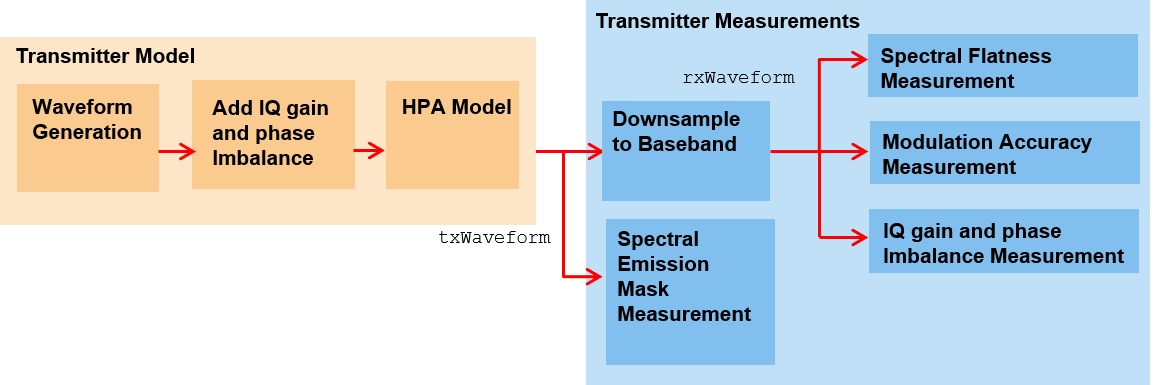### 802.11ac VHT Packet Configuration

This example generates an IEEE 802.11ac waveform consisting of multiple VHT format packets. Use the VHT format configuration object, `wlanVHTConfig`, to configure transmission properties of a VHT packet. This example configures the VHT waveform for a 80 MHz bandwidth. Because this example does not use space-time block coding, it can measure the modulation accuracy per spatial stream.

```cfgVHT = wlanVHTConfig; % Create packet configuration cfgVHT.ChannelBandwidth = 'CBW80'; % 80 MHz cfgVHT.NumTransmitAntennas = 1; % One transmit antenna cfgVHT.NumSpaceTimeStreams = 1; % One space-time stream cfgVHT.STBC = false; % No STBC so one spatial stream cfgVHT.MCS = 8; % Modulation: 256-QAM cfgVHT.APEPLength = 3000; % A-MPDU length pre-EOF padding in bytes```

### Waveform Generation

Generate the VHT waveform for the specified bits and configuration by using the `wlanWaveformGenerator` function, specifying the desired oversampling factor, number of packets, and idle time between each packet.

```osf = 3; % 3x oversampling factor numPackets = 20; % Generate 20 packets idleTime = 10e-6; % 10 microseconds idle time between packets```

Create random bits for all packets, `data`, and pass as an argument to `wlanWaveformGenerator` along with the VHT packet configuration object `cfgVHT`. This configures the waveform generator to synthesize an 802.11ac VHT waveform. Additionally, configure the waveform generator by using name-value pairs to generate multiple oversampled packets with a specified idle time between each packet.

```% Create random data; PSDULength is in bytes savedState = rng(0); % Set random state data = randi([0 1],cfgVHT.PSDULength*8*numPackets,1); % Generate a multi-packet waveform txWaveform = wlanWaveformGenerator(data,cfgVHT, ... 'OversamplingFactor',osf,'NumPackets',numPackets,'IdleTime',idleTime); fs = wlanSampleRate(cfgVHT); % Baseband sampling rate of the waveform```

#### IQ Imbalance Modeling

IQ imbalance arises when a front-end component does not respect the power balance or the orthogonality between the I and Q branches. This example adds IQ gain and phase imbalance to the transmitted waveform based on the flag `modelIQImbalance`. At the receiver, estimate the IQ gain and phase imbalance and correct the waveform as per the compensation scheme specified in [ 5 ].

```modelIQImbalance = true; % Set to true to add IQ gain and phase imbalance if modelIQImbalance iqGaindB = 1; % IQ gain imbalance in dB, specify from the range [-1 1] iqPhaseDeg = 1; % IQ phase imbalance in degrees, specify from the range [-2 2] iqGainLin = db2mag(iqGaindB); % Convert gain from dB to linear value txWaveform = real(txWaveform) + 1i*imag(txWaveform)*iqGainLin*exp(1j*iqPhaseDeg*pi/180); % As specified in Equation-1 of  end```

#### High-Power Amplifier Modeling

The high-power amplifier introduces nonlinear behavior in the form of inband distortion and spectral regrowth. This example simulates the power amplifiers by using the Rapp model in 802.11ac [ 2 ], which introduces AM/AM distortion.

Model the amplifier by using the `comm.MemorylessNonlinearity` object, and configure reduced distortion by specifying a backoff, `hpaBackoff`, such that the amplifier operates below its saturation point. You can increase the backoff to reduce EVM for higher MCS values.

```pSaturation = 25; % Saturation power of a power amplifier in dBm hpaBackoff = 13; % Power amplifier backoff in dB % Create and configure a memoryless nonlinearity to model the amplifier nonLinearity = comm.MemorylessNonlinearity; nonLinearity.Method = 'Rapp model'; nonLinearity.Smoothness = 3; % p parameter nonLinearity.LinearGain = -hpaBackoff; nonLinearity.OutputSaturationLevel = db2mag(pSaturation-30); % Apply the model to each transmit antenna txWaveform = nonLinearity(txWaveform);```

#### Thermal Noise

Add thermal noise to each transmit antenna by using the `comm.ThermalNoise` object with a noise figure of 6 dB [ 3 ].

```thNoise = comm.ThermalNoise('NoiseMethod','Noise Figure','SampleRate',fs*osf,'NoiseFigure',6); for i = 1:cfgVHT.NumTransmitAntennas txWaveform(:,i) = thNoise(txWaveform(:,i)); end```

### Modulation Accuracy (EVM), Spectral Flatness and IQ Imbalance Measurements

#### Downsampling and Filtering

Resample the oversampled waveform down to baseband for physical layer processing and EVM and spectral flatness measurements, applying a low-pass anti-aliasing filter before downsampling. The impact of the low-pass filter is visible in the spectral flatness measurement. The anti-aliasing filter is designed so that all active subcarriers are within the filter passband.

Design resampling filter.

```aStop = 40; % Stopband attenuation ofdmInfo = wlanVHTOFDMInfo('VHT-Data',cfgVHT); % OFDM parameters SCS = fs/ofdmInfo.FFTLength; % Subcarrier spacing txbw = max(abs(ofdmInfo.ActiveFrequencyIndices))*2*SCS; % Occupied bandwidth [L,M] = rat(1/osf); maxLM = max([L M]); R = (fs-txbw)/fs; TW = 2*R/maxLM; % Transition width firdec = designMultirateFIR(L,M,TW,aStop,'SystemObject',true);```

Resample the waveform to baseband.

`rxWaveform = firdec(txWaveform);`

This section detects, synchronizes, and extracts each packet in `rxWaveform`, then measures the EVM, spectral flatness, and IQ imbalance. For each packet, the example performs these steps:

• Detect the start of the packet

• Extract the non-HT fields

• Estimate and correct coarse carrier frequency offset (CFO)

• Perform fine symbol timing estimate by using the frequency-corrected non-HT fields

• Extract the packet from the waveform by using the fine symbol timing offset

• Correct the extracted packet with the coarse CFO estimate

• Extract the L-LTF, then estimate the fine CFO and correct for the whole packet

• Extract the VHT-LTF and perform channel estimation for each of the transmit streams

• Measure the IQ imbalance from the channel estimate and perform correction on the channel estimate

• Measure the spectral flatness by using the channel estimate

• Extract and OFDM demodulate the VHT data field

• Perform noise estimation by using the demodulated data field pilots and single-stream channel estimate at pilot subcarriers

• Phase-correct and equalize the VHT data field by using the channel and noise estimates

• Correct the equalized data subcarriers with the IQ imbalance estimate

• For each data-carrying subcarrier in each spatial stream, find the closest constellation point and measure the EVM

The diagram shows the processing chain: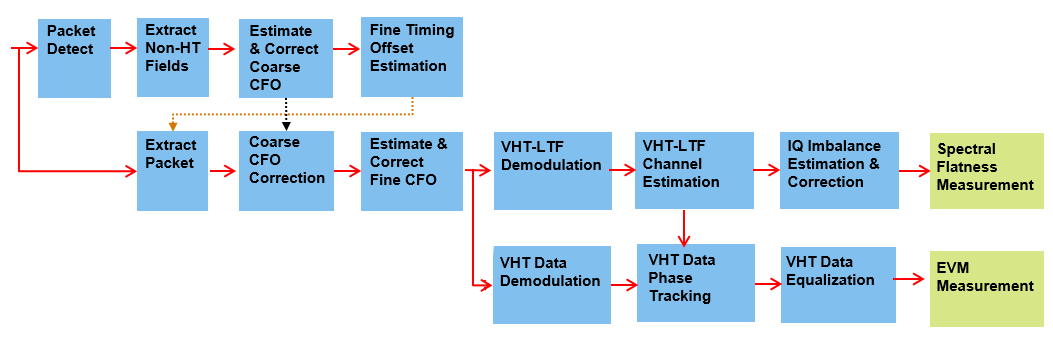The VHT-LTF symbols include pilot symbols to allow for phase tracking, but this example does not perform phase tracking.

Test the spectral flatness for each packet by measuring the deviation in the magnitude of individual subcarriers in the channel estimate against the average [ 1 ]. Plot these deviations for each packet using the helper function vhtTxSpectralFlatnessMeasurement. Plot the average EVM per data-carrying subcarrier and the equalized symbols for each packet.

Demodulate, equalize, and decode the VHT Data symbols by using the `wlanVHTDataRecover` function. Parameterize this function to perform pilot phase tracking and zero-forcing equalization as required by the standard. This example measures the modulation accuracy from the equalized symbols.

This example makes two different EVM measurements using two instances of `comm.EVM`.

• RMS EVM per packet, which comprises averaging the EVM over subcarriers, OFDM symbols, and spatial streams.

• RMS EVM per subcarrier per spatial stream for a packet. Because this configuration maps spatial streams directly to antennas, this measurement can help detect frequency-dependent impairments, which may affect individual RF chains differently. This measurement averages the EVM over OFDM symbols only.

```% Setup EVM measurements [EVMPerPkt,EVMPerSC] = vhtEVMSetup(cfgVHT);```

This code configures objects and variables for processing.

```% Get indices for accessing each field within the time-domain packet ind = wlanFieldIndices(cfgVHT); rxWaveformLength = size(rxWaveform,1); pktLength = double(ind.VHTData(2)); % Define the minimum length of data we can detect; length of the L-STF in % samples minPktLen = double(ind.LSTF(2)-ind.LSTF(1))+1; % Setup the measurement plots [hSF,hCon,hEVM] = vhtTxSetupPlots(cfgVHT); rmsEVM = zeros(numPackets,1); pktOffsetStore = zeros(numPackets,1); rng(savedState); % Restore random state```

Detect and process packets within the received waveform, `rxWaveform` by using a while loop, which performs these steps.

• Detect a packet by indexing into `rxWaveform` with the sample offset, `searchOffset`

• Detect and process the first packet within `rxWaveform`

• Detect and process the next packet by incrementing the sample index offset, `searchOffset`

• Repeat until no further packets are detected

```pktNum = 0; searchOffset = 0; % Start at first sample (no offset) while (searchOffset+minPktLen)<=rxWaveformLength % Packet detect pktOffset = wlanPacketDetect(rxWaveform,cfgVHT.ChannelBandwidth); % Packet offset from start of waveform pktOffset = searchOffset+pktOffset; % If no packet detected or offset outwith bounds of waveform then stop if isempty(pktOffset) || (pktOffset<0) || ... ((pktOffset+ind.LSIG(2))>rxWaveformLength) break; end % Extract non-HT fields and perform coarse frequency offset correction % to allow for reliable symbol timing nonht = rxWaveform(pktOffset+(ind.LSTF(1):ind.LSIG(2)),:); coarsefreqOff = wlanCoarseCFOEstimate(nonht,cfgVHT.ChannelBandwidth); nonht = frequencyOffset(nonht,fs,-coarsefreqOff); % Determine offset between the expected start of L-LTF and actual start % of L-LTF lltfOffset = wlanSymbolTimingEstimate(nonht,cfgVHT.ChannelBandwidth); % Determine packet offset pktOffset = pktOffset+lltfOffset; % If offset is without bounds of waveform skip samples and continue % searching within remainder of the waveform if (pktOffset<0) || ((pktOffset+pktLength)>rxWaveformLength) searchOffset = pktOffset+double(ind.LSTF(2))+1; continue; end % Timing synchronization complete; extract the detected packet rxPacket = rxWaveform(pktOffset+(1:pktLength),:); pktNum = pktNum+1; disp([' Packet ' num2str(pktNum) ' at index: ' num2str(pktOffset+1)]); % Apply coarse frequency correction to the extracted packet rxPacket = frequencyOffset(rxPacket,fs,-coarsefreqOff); % Perform fine frequency offset correction on the extracted packet lltf = rxPacket(ind.LLTF(1):ind.LLTF(2),:); % Extract L-LTF fineFreqOff = wlanFineCFOEstimate(lltf,cfgVHT.ChannelBandwidth); rxPacket = frequencyOffset(rxPacket,fs,-fineFreqOff); % Extract VHT-LTF samples, demodulate and perform channel estimation vhtltf = rxPacket(ind.VHTLTF(1):ind.VHTLTF(2),:); vhtltfDemod = wlanVHTLTFDemodulate(vhtltf,cfgVHT); % Get single stream channel estimate chanEstSSPilots = vhtSingleStreamChannelEstimate(vhtltfDemod,cfgVHT); % Channel estimate chanEst = wlanVHTLTFChannelEstimate(vhtltfDemod,cfgVHT); % Perform IQ gain and phase imbalance estimation [gainEst,phaseEst,alphaEst,betaEst,gamma,dataRot] = ... helperIQImbalanceEstimate(chanEst,cfgVHT); fprintf(' Measured IQ gain & phase imbalance: %2.2f dB, %2.2f deg\n',gainEst,phaseEst); % Perform IQ gain and phase imbalance correction on channel % estimates chanEst = chanEst./(alphaEst + betaEst.*gamma); % As specified in Equation-29 of  % Spectral flatness test [pass,deviation,testsc] = wlanSpectralFlatness(chanEst,'VHT',cfgVHT.ChannelBandwidth); if pass disp(' Spectral flatness passed'); else disp(' Spectral flatness failed'); end % Plot deviation against limits vhtTxPlotSpectralFlatness(deviation,testsc,pktNum,hSF); % Extract VHT Data samples from the waveform vhtdata = rxPacket(ind.VHTData(1):ind.VHTData(2),:); % Estimate the noise power in VHT data field noiseVarVHT = vhtNoiseEstimate(vhtdata,chanEstSSPilots,cfgVHT); % Extract VHT Data samples and perform OFDM demodulation, equalization % and phase tracking [~,~,eqSym] = wlanVHTDataRecover(vhtdata,chanEst,noiseVarVHT,cfgVHT,... 'EqualizationMethod','ZF','PilotPhaseTracking','PreEQ','LDPCDecodingMethod','norm-min-sum'); % Use zero forcing algorithm for equalization % Perform IQ gain and phase imbalance correction on VHT data eqSym = eqSym.*dataRot; % Carrier rotation on data subcarriers eqSym = ((conj(alphaEst)*eqSym)-(betaEst*conj(eqSym(end:-1:1,:,:))))/((abs(alphaEst)^2)-(abs(betaEst)^2)); % As specified in Equation-30 of  % Compute RMS EVM over all spatial streams for packet rmsEVM(pktNum) = EVMPerPkt(eqSym); fprintf(' RMS EVM: %2.2f%%, %2.2fdB\n',rmsEVM(pktNum),20*log10(rmsEVM(pktNum)/100)); % Compute RMS EVM per subcarrier and spatial stream for the packet evmPerSC = EVMPerSC(eqSym); % Nst-by-1-by-Nss % Plot RMS EVM per subcarrier and equalized constellation vhtTxEVMConstellationPlots(eqSym,evmPerSC,cfgVHT,pktNum,hCon,hEVM); % Store the offset of each packet within the waveform pktOffsetStore(pktNum) = pktOffset; % Increment waveform offset and search remaining waveform for a packet searchOffset = pktOffset+pktLength+minPktLen; end```
``` Packet 1 at index: 25 ```
``` Measured IQ gain & phase imbalance: 0.98 dB, 0.98 deg ```
``` Spectral flatness passed ```
``` RMS EVM: 3.30%, -29.63dB ```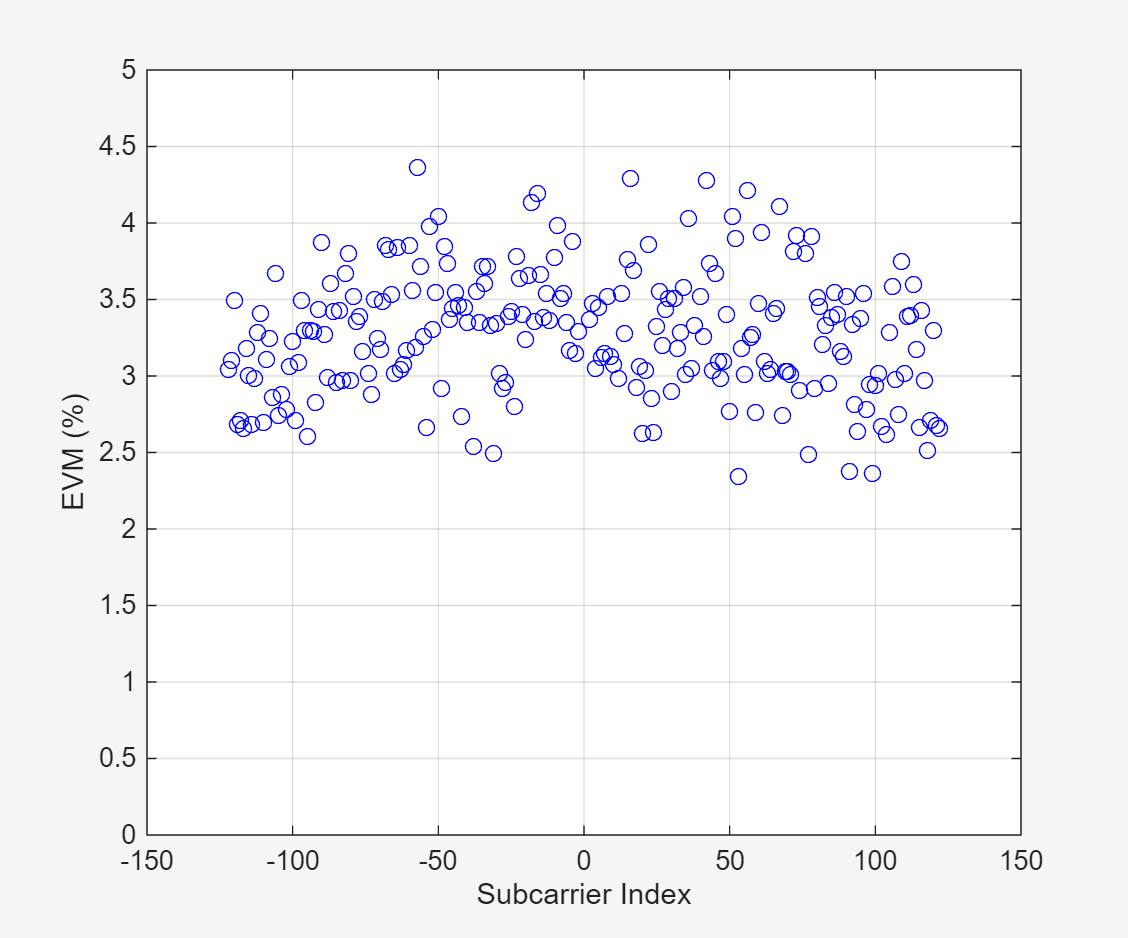``` Packet 2 at index: 9785 ```
``` Measured IQ gain & phase imbalance: 0.98 dB, 0.98 deg ```
``` Spectral flatness passed `````` RMS EVM: 3.07%, -30.24dB ```
``` Packet 3 at index: 19545 ```
``` Measured IQ gain & phase imbalance: 0.98 dB, 0.98 deg ```
``` Spectral flatness passed ```
``` RMS EVM: 2.91%, -30.71dB ```
``` Packet 4 at index: 29305 ```
``` Measured IQ gain & phase imbalance: 0.98 dB, 0.98 deg ```
``` Spectral flatness passed ```
``` RMS EVM: 3.04%, -30.33dB ```
``` Packet 5 at index: 39065 ```
``` Measured IQ gain & phase imbalance: 0.98 dB, 0.98 deg ```
``` Spectral flatness passed ```
``` RMS EVM: 2.99%, -30.50dB ```
``` Packet 6 at index: 48825 ```
``` Measured IQ gain & phase imbalance: 0.98 dB, 0.98 deg ```
``` Spectral flatness passed ```
``` RMS EVM: 2.67%, -31.49dB ```
``` Packet 7 at index: 58585 ```
``` Measured IQ gain & phase imbalance: 0.98 dB, 0.98 deg ```
``` Spectral flatness passed ```
``` RMS EVM: 2.93%, -30.65dB ```
``` Packet 8 at index: 68345 ```
``` Measured IQ gain & phase imbalance: 0.98 dB, 0.98 deg ```
``` Spectral flatness passed ```
``` RMS EVM: 2.81%, -31.03dB ```
``` Packet 9 at index: 78105 ```
``` Measured IQ gain & phase imbalance: 0.98 dB, 0.98 deg ```
``` Spectral flatness passed ```
``` RMS EVM: 2.93%, -30.65dB ```
``` Packet 10 at index: 87865 ```
``` Measured IQ gain & phase imbalance: 0.98 dB, 0.98 deg ```
``` Spectral flatness passed ```
``` RMS EVM: 2.73%, -31.28dB ```
``` Packet 11 at index: 97625 ```
``` Measured IQ gain & phase imbalance: 0.98 dB, 0.98 deg ```
``` Spectral flatness passed ```
``` RMS EVM: 2.90%, -30.76dB ```
``` Packet 12 at index: 107385 ```
``` Measured IQ gain & phase imbalance: 0.98 dB, 0.98 deg ```
``` Spectral flatness passed ```
``` RMS EVM: 2.94%, -30.63dB ```
``` Packet 13 at index: 117145 ```
``` Measured IQ gain & phase imbalance: 0.98 dB, 0.98 deg ```
``` Spectral flatness passed ```
``` RMS EVM: 2.84%, -30.94dB ```
``` Packet 14 at index: 126905 ```
``` Measured IQ gain & phase imbalance: 0.98 dB, 0.98 deg ```
``` Spectral flatness passed ```
``` RMS EVM: 2.91%, -30.73dB ```
``` Packet 15 at index: 136665 ```
``` Measured IQ gain & phase imbalance: 0.98 dB, 0.98 deg ```
``` Spectral flatness passed ```
``` RMS EVM: 3.09%, -30.20dB ```
``` Packet 16 at index: 146425 ```
``` Measured IQ gain & phase imbalance: 0.98 dB, 0.98 deg ```
``` Spectral flatness passed ```
``` RMS EVM: 2.60%, -31.69dB ```
``` Packet 17 at index: 156185 ```
``` Measured IQ gain & phase imbalance: 0.98 dB, 0.98 deg ```
``` Spectral flatness passed ```
``` RMS EVM: 2.99%, -30.49dB ```
``` Packet 18 at index: 165945 ```
``` Measured IQ gain & phase imbalance: 0.98 dB, 0.98 deg ```
``` Spectral flatness passed ```
``` RMS EVM: 3.20%, -29.90dB ```
``` Packet 19 at index: 175705 ```
``` Measured IQ gain & phase imbalance: 0.98 dB, 0.98 deg ```
``` Spectral flatness passed ```
``` RMS EVM: 3.24%, -29.79dB ```
``` Packet 20 at index: 185465 ```
``` Measured IQ gain & phase imbalance: 0.98 dB, 0.98 deg ```
``` Spectral flatness passed ```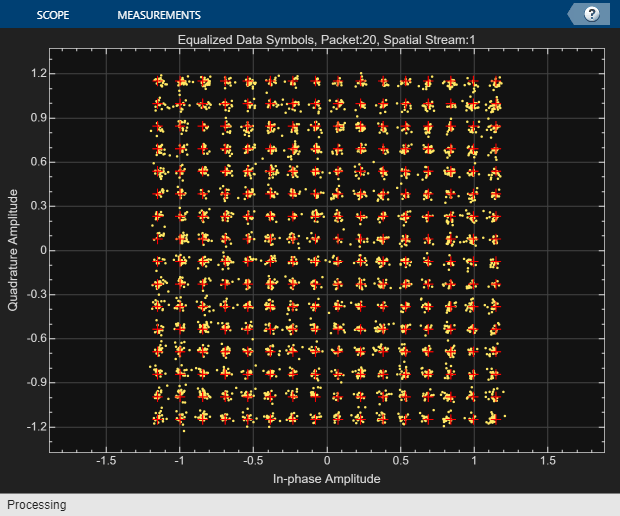``` RMS EVM: 3.20%, -29.91dB ```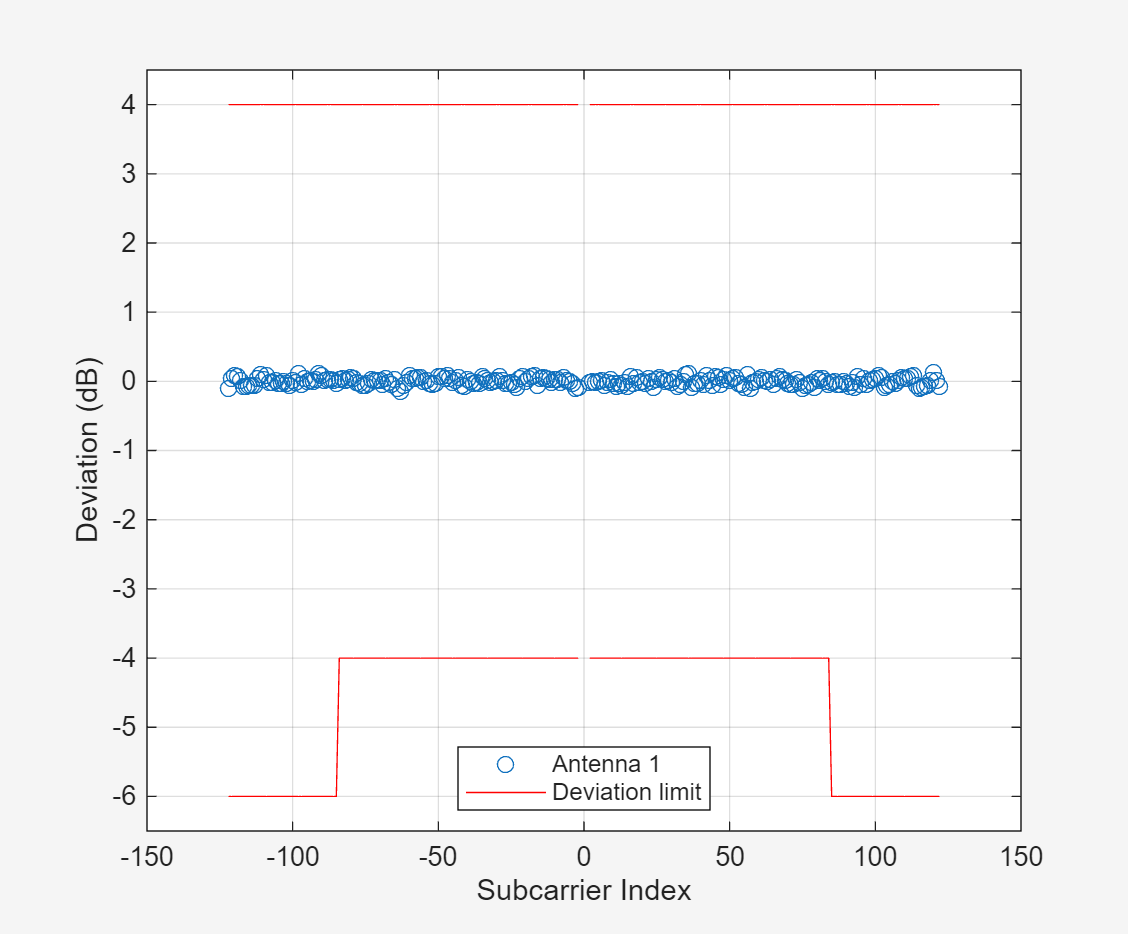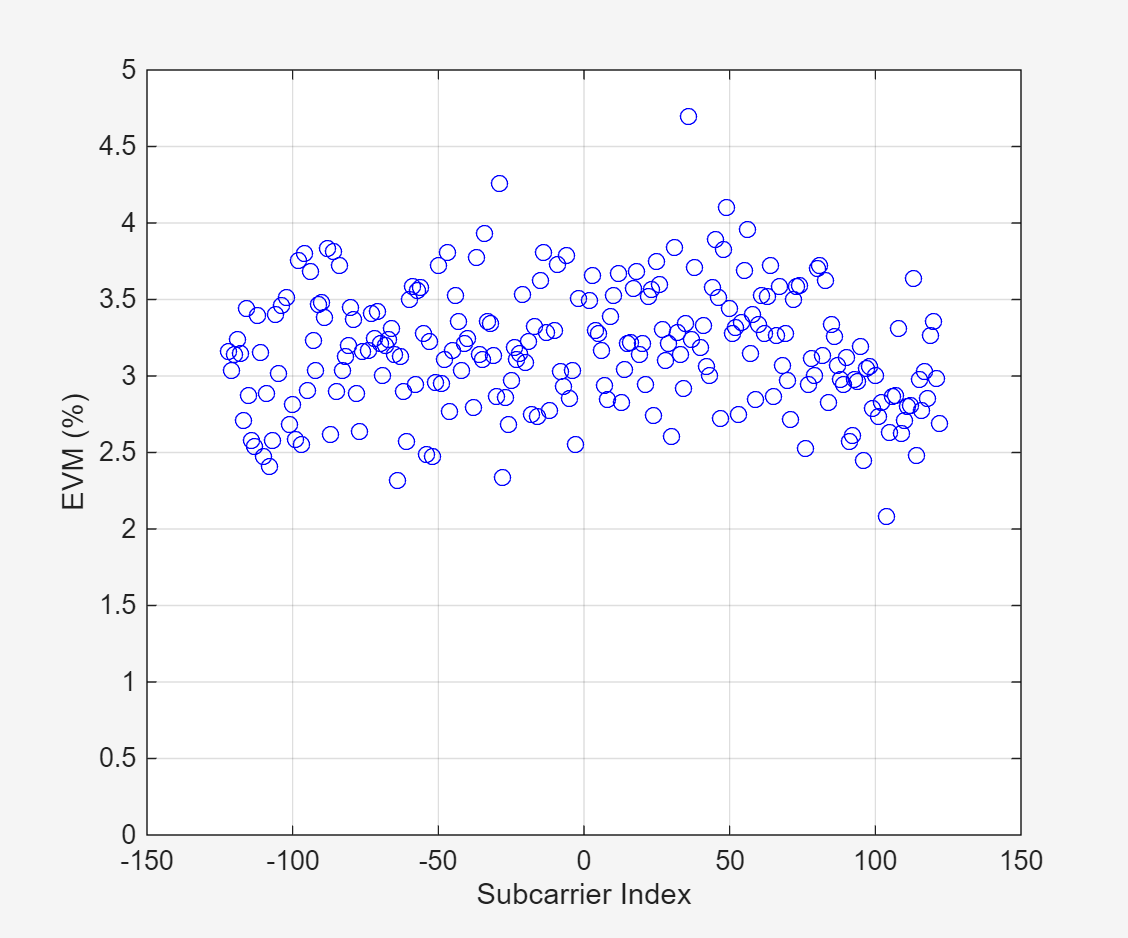```if pktNum>0 fprintf('Average EVM for %d packets: %2.2f%%, %2.2fdB\n', ... pktNum,mean(rmsEVM(1:pktNum)),20*log10(mean(rmsEVM(1:pktNum))/100)); else disp('No complete packet detected'); end```
```Average EVM for 20 packets: 2.96%, -30.56dB ```

### Transmit Spectrum Emission Mask Measurement

This section measures the spectral mask of the filtered and impaired waveform after high-power amplifier modeling.

The transmitter spectral mask test [ 4 ] uses a time-gated spectral measurement of the VHT Data field. The example extracts the VHT Data field of each packet from the oversampled waveform, `txWaveform`, by using the start indices of each packet within the waveform. Any delay introduced in the baseband processing chain used to determine the packet indices must be accounted for when gating the VHT data field within `txWaveform`. Concatenate the extracted VHT Data fields in preparation for measurement.

```% Indices for accessing each field within the time-domain packet ind = wlanFieldIndices(cfgVHT,'OversamplingFactor', osf); startIdx = ind.VHTData(1); % Start of Data endIdx = ind.VHTData(2); % End of Data idleNSamps = idleTime*fs*osf; % Idle time samples perPktLength = endIdx+idleNSamps; idx = zeros(endIdx-startIdx+1,numPackets); for i = 1:numPackets % Start of packet in txWaveform, accounting for the filter delay pktOffset = (i-1)*perPktLength; % Indices of non-HT Data in txWaveform idx(:,i) = pktOffset+(startIdx:endIdx); end gatedVHTData = txWaveform(idx(:),:);```

The 802.11ac standard specifies the spectral mask relative to the peak power spectral density. The helper function helperSpectralMaskTest generates a plot which overlays the required mask with the measured PSD.

```if pktNum>0 helperSpectralMaskTest(gatedVHTData,fs,osf); end```
``` Spectrum mask passed ```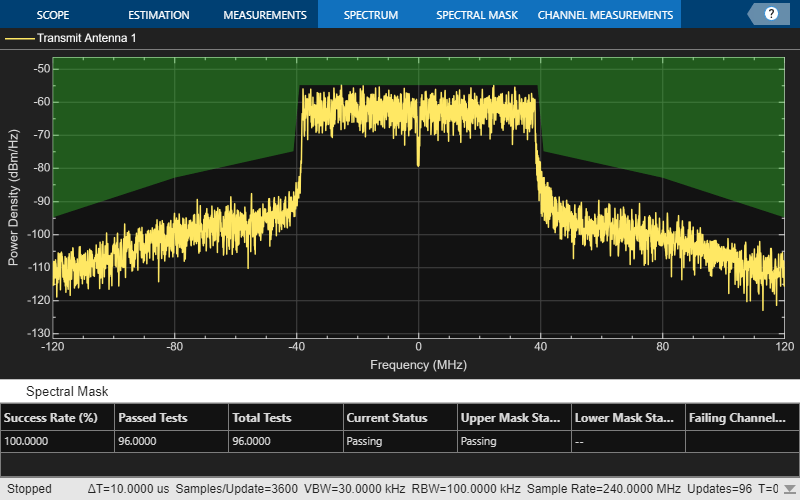### Conclusion and Further Exploration

This example plots four results: spectral flatness, RMS EVM per subcarrier, equalized constellation, and spectral mask.

The high-power amplifier model introduces significant inband distortion and spectral regrowth which is visible in the EVM results, noisy constellation and out-of-band emissions in the spectral mask plot. Try increasing the high-power amplifier backoff and note the improved EVM, constellation and lower out-of-band emissions.

Try using different values for `iqGaindB` and `iqPhaseDeg` and note the impact on EVM and constellation.

Related examples:

### Selected Bibliography

1. IEEE Std 802.11™-2020 IEEE Standard for Information Technology - Telecommunications and Information Exchange Between Systems - Local and Metropolitan Area Networks - Specific Requirements - Part 11: Wireless LAN Medium Access Control (MAC) and Physical Layer (PHY) Specifications.

2. Loc and Cheong. IEEE P802.11 Wireless LANs. TGac Functional Requirements and Evaluation Methodology Rev. 16. 2011-01-19.

3. Perahia, E., and R. Stacey. Next Generation Wireless LANs: 802.11n and 802.11ac. 2nd Edition. United Kingdom: Cambridge University Press, 2013.

4. Archambault, Jerry, and Shravan Surineni. "IEEE 802.11 spectral measurements using vector signal analyzers." RF Design 27.6 (2004): 38-49.

5. M. Janaswamy, N. K. Chavali and S. Batabyal, "Measurement of transmitter IQ parameters in HT and VHT wireless LAN systems," 2016 International Conference on Signal Processing and Communications (SPCOM), Bangalore.